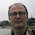## Pages

### Hackerrank Hash Tables: Ransom Note

Given two lists of words, we have to say if the second is a subset of the first. This HackerRank problem is very similar to the previous one.

The given sample, that I converted to a python test, should clarify the point of the problem:
```def test_provided_1(self):
self.assertEqual(True, solution(['give', 'me', 'one', 'grand', 'today', 'night'], ['give', 'one', 'grand', 'today']))
```
I don't bother to add more tests, being the problem so simple. And I don't even spend much time in thinking on the function design, since the problem name itself gives a huge spoiler on its solution. I would put the words in hash tables (dictionaries, since I am using python as implementation language) and I ensure all the words in the second collection are also present in the first one.
```from collections import Counter  # 1

def solution(magazine, ransom):
available = Counter(magazine)
needed = Counter(ransom)  # 2

for word in needed:
if needed.get(word) > available.get(word, 0):  # 3
return False
return True
```
1. Since it is all about counting items, instead of using naked dictionaries I use Counters, making my script a bit shorter. See my solution to the previous problem to see how the code looks like using dictionaries.
2. Actually, the second dictionary is not strictly required, and a check as implemented in the previous problem would reduce slightly the cost of the algorithm. However, I am not here to write optimized code (otherwise I would have chosen C++ as implementation language), and in this way the code is probably more readable.
3. If I don't have enough of the current word, or even not at all, I can stop looping and return a False. Only if all the words in ransom are also in magazine I'll return True.

Solution accepted, unit test and python script pushed to GitHub.

1.what would the big O complexity be?

1.This algorithm has linear time complexity with respect to the biggest among N and M, being them the sizes of the two lists in input.

The construction complexity of a Counter is linear on the size of the passed list, since we have to scan all the element in the list.
The for loop is linear on the size of ransom, since the get() cost on a hash table is constant in time.

Adding up the linear complexity of the three blocks we end up with linear complexity.

Notice that if there are just a handful of words in the ransom note and the magazine word list is huge, the most expensive line in the algorithm is number 5, the available Counter creation.

Have I answered to your question? Is it more clear now?